Perceptions of Physical Reality - 2 by Raj Vatsya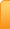Channels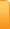Analysis
Cartoons
Education
Environment
Opinion
Photo Essays

Random Thoughts

Architecture
Astrology
Ayurveda
Buddhism
Cinema
Culture
Festivals
Hinduism
History
People
Places
Sikhism
Spirituality

Health
Parenting
Perspective
Recipes
Society
Teens
Women

## Creative Writings

Book Reviews
Computing
Humor
Individuality
Literary Shelf
Memoirs
Quotes
Stories
Travelogues
Workshop

Perceptions of Physical Reality - 2
by Dr. Raj Vatsya

Classical Theories - Motion of Particles

I had given an overview of the human perceptions of physical reality from the earliest times the matter was systematically studied to the present in Perceptions of Physical Reality – An Overview, cited as [I] here, which provides the background for the present article that should be checked for explanations of some concepts appearing here, even if it is not explicitly cited.Most of physics is centered about the motion of particles together with the necessary related concepts. The present article describes our pertaining understanding starting with the time of Kepler and Galileo, from the late sixteenth century to early seventeenth, to the end of the nineteenth century with the relativistic developments made during an early period of the twentieth century included, which is now known as the classical physics. In 1900 Planck noticed that light was quantized as particles, which was the beginning of the quantum theories. Development of the theory of relativity was completed towards the end of the second decade of the twentieth century, which is included in the classical physics.

Preliminaries

In the classical thinking, reality is considered to be objective and an observer’s role is only to decipher it, which is the “realist” view. I am using the term “realist” referring to “realism” in understanding nature [I] to avoid confusion, which may result from use of the term “realistic” due to its usual connotation. Physical systems in general together with the pertaining concepts are understood on the background of mathematical structures by formulating the physical concepts in terms of the mathematical ones, which establishes a correspondence between the two. Since the purpose of physics is to describe reality and mathematics is a construct of the human mind, which by itself has nothing to do with reality, the process of understanding reality constitutes of mapping it into a construct of the mind. It would appear therefore that a formulation of an understanding, i.e., a theory, is not independent of the mind; mind puts something in it; thus different minds can come up with different understandings. However, some authors insist that an understanding of reality is as objective as the reality itself, in order to adhere completely to the realist view, which would otherwise be compromised for the understanding of reality is all the reality we can know. According to this realist view, all apparently differing correct theories must be equivalent to each other.

To construct a mathematical structure, one needs to understand a variety of concepts, which are described in terms of certain others, which need be defined in turn. This process by itself is circular defining nothing. In mathematics, this cycle is broken by considering some concepts to be primal, self-evident, inherently self-defined. Also, as discussed in [I], a mathematical structure is built upon a self-consistent set of assumptions termed the axioms, which themselves are essentially primal in nature.

Euclidean Geometry

The processes of nature take place, or are understood, on the setting of the space-time complex. In the classical physics, the motion of a particle entails tracking its location in space with respect to time. The space is considered to be an absolute non-material relational extent, in the sense that it exists independent of the time and observer. Time is measured in terms of the locations of some moving object, e.g., the needle of a clock. Thus tracking the motion is essentially a calibration of the locations of two moving bodies.

The way space is defined here cognizes it essentially in terms of the isolated points, with “point” being a primal. Understanding the concept of motion requires connecting the points by a line in an unambiguous way, which requires an introduction of a geometrical structure in the space. In the classical theories the geometry of space is taken to be the Euclidean geometry, which is founded upon five axioms, postulates. We mention three of them here: One can draw a unique straight line from one point to the other; a finite straight line can be extended further in a straight line; and all right angles are equal to each other.

Euclid defines the right angle as follows:

“When a straight line set up on a straight line makes the adjacent angles equal to one another, each of the equal angles is right, and the straight line standing on the other is called a perpendicular to that on which it stands.”

It may appear that we are complicating things unnecessarily by formalizing something so obvious. However, we had learned early on that to develop a consistent mathematical system, we must formulate it on the foundation of a set of self-consistent axioms. That some concepts are “obvious,” renders those all the more suitable to be taken as axioms, but relying on such intuition based perceptions is not safe. In fact slight alterations to axioms have yielded drastically different structures, some of which are used in constructing the theories in physics.

Given the axioms of the Euclidean geometry, one can take a point in space and draw a straight line from it; then one can draw another straight line from the same point at a right angle to the first; and a third one at right angles to both of the previous ones. The common point is the origin and three lines are usually called the x, y and z axis. This formalizes our common perception of the three-dimensional space around us and introduces this, the Cartesian, coordinate system in it. Every point in space can be identified by a set of three distances, each along an axis from the origin. Distances are measured by comparing them with an arbitrarily selected rigid measuring rod, e.g., the meter. We can now track the motion of a particle by identifying its location with respect to the time, treated as an independent parameter, in this structure termed a frame of reference.

Motion along a continuing straight line can be tracked as above as it can be obtained from a straight line by extending it indefinitely. Motion along the lines that are not straight can be tracked by determining the location at many time points close to each other and connect the consecutive points with straight lines; then we would have a clearer picture of the trajectory, which the particle follows. This picture can be refined by making the time-mesh finer and finer. In the limit of the time intervals tending to zero, we would have the exact picture. Such limiting procedures are mathematically legitimate, rigorous, and among the most useful constructions in mathematics including wherever mathematical methods are used, e.g., physics. Incidentally, we have also defined a curved line as the limit of a continuing union of the piece-wise straight lines.

Consider a particle moving in a straight line with the origin as one of the points on it. Its speed is now defined as the rate of change of its distance from the origin with respect to time. Velocity is the speed and direction put together. Thus, a particle travelling eastward with a constant speed has different velocity from an identical particle travelling northwards with the same speed. Velocity is identified by three numbers, i.e., the speeds along three axes. Acceleration is defined to be the rate of change of the velocity, again identified by three numbers. Thus, we have defined various concepts related to motion in a fixed frame of reference in terms the axioms of the Euclidean geometry. Motion in moving frames of reference is considered next.

Principle of Relativity

Galileo theorized that the laws of physics are the same in all frames of reference moving at constant velocities with respect to each other. Such frames of reference are called the inertial frames of reference. This is known as Galileo’s principle of relativity.

To illustrate:

Consider an observer in a train moving along a straight track at a constant speed with respect to the ground. The reference frames attached to the ground and the train, are inertial with respect to each other. If an observer in the train releases a ball in the air, it would fall to the floor the same way as it would if released above the ground by an observer on the ground. If the ball is propelled horizontally at a constant speed, the trajectory it would travel along would be identical to the one it would travel along if it was propelled with the same velocity on the ground. According to Galileo’s principle of relativity, no experiment can distinguish one inertial frame from the other, i.e., the laws determining the outcome of an experiment are the same in both.

Although the laws are the same in two inertial frames, the observations of the same phenomenon from two different inertial frames can differ. For example, if a ball is released in air inside the train, the observer inside the train would observe it falling to the ground vertically; but the observer on the ground would observe it taking the same trajectory as a ball propelled on the ground with the velocity the train is moving at. The rules to relate the locations and the time and thus, the observations, in two inertial frames are called the Galilean transformations explained below.

The time measurements are the same in all frames and the length of a rigid rod also remains constant regardless of the frame. According to the Galilean transformation rules, the velocities and distances measured in different inertial frames are calculated as follows. Consider the case of the train moving at 100 km/hr with respect to the ground. Denote the frame of reference attached to the ground by A. Suppose another train moving at 70 km/hr is following it; denote the frame attached to this train by B. The frames A and B are inertial with respect to each other. While the velocity of the first train in frame A is 100 km/hr, it is 30 km/hr in frame B. Distances are calculated by multiplying the velocity with the time lapsed. Galileo’s principle of relativity then means: If the distances and time points are transformed according to the Galilean transformation rules, the laws of physics would assume the same form in all inertial frames.

This formulation of the principle of relativity was a radical departure from the earlier thinking, and it provided the foundation for the pertaining thinking in later times, so much so that it is a part of our common thinking now as should be clear from the example of the moving trains used above.

Laws of Motion

Modern classical understanding of the particle motion began with the studies of planetary motion. The planets were idealized as point particles orbiting around the sun, also assumed to be concentrated in a point. Ignoring the extension of the bodies is legitimate if it is small compared to the length of trajectory under consideration, e.g., the planets and the sun are miniscule compared to the extent of a planet’s orbit. Such idealizations are standard in physics; deviations from an idealized system are considered as perturbations if they become significant. Based on a large body of observations, Kepler formulated what are known as Kepler’s laws of planetary motion. According to Kepler’s first law, the planetary orbits are ellipses; and according to the second, the line joining a planet and the Sun sweeps out equal areas during equal intervals of time. The third law relates the orbital period with the major axis of the ellipse. The scope of these laws is limited to describing the motion of bodies with the same properties and in the same configurations as the planets. As explained in [I], these laws are therefore empirical in nature, while the aim in physics is to formulate the observations upon the laws of fundamental nature. However, these laws together with Galileo’s observations and theories led Newton to formulate his law of gravitation and three laws of motion, which possess a fundamental character. As Newton commented, “I have been able to see farther for I stood on the shoulders of giants like Kepler and Galileo.” We shall return to the matters related to gravitation in the next article in this series. Here we discuss the laws of particle motion.

• First law: “Everybody persists in its state of being at rest or of moving uniformly straight forward, except insofar as it is compelled to change its state by force impressed.” Equivalently: A body moves with a constant velocity unless acted upon by a force. The state of being at rest is included in this statement for it corresponds to the zero velocity.

• Second law: “The alteration of motion is ever proportional to the motive force impressed; and is made in the direction of the right line in which that force is impressed.”

This original statement, a translation from Latin as are others, is not clear in itself. The lack of clarity comes from the term “alteration of motion.” If “alteration of motion” is taken to be the rate of change of velocity, i.e., acceleration, then the law states that the acceleration produced in a body by a force impressed upon it is proportional to the force. The constant of proportionally was determined to be the mass of body, adjusting the law to: Mass multiplied by acceleration is equal to the force. This is how the law is understood now.

• Third law: “To every action there is always an equal and opposite reaction; or the forces of two bodies on each other are always equal and are directed in opposite directions.” That is to say: When one body exerts a force on a second body, the second body simultaneously exerts a force equal in magnitude and opposite in direction to that of the first body.

These statements of Newton’s laws of motion do not refer explicitly to an inertial frame. However, supported by the related discourse, the laws are assumed to hold in an inertial frame of reference. Newton’s definition of an inertial frame was the one that moves with a constant velocity with respect to the fixed stars. Objections were raised against this concept of the inertial frames mainly because it goes against the internal logic of the classical mechanics in that according to Galileo’s principle of relativity, which Newton accepted, no inertial frame of reference can be distinguished from the other and thus the frame attached to the fixed stars cannot be singled out, if there could be any fixed stars. Some authors define the inertial frames as the ones where the first law holds, providing the grounds for the other laws. The first law is then assumed to state that if the law holds in a frame of reference, then it is an inertial frame; and thus, the law holds in another one moving with a constant velocity with respect to this one. However, the other laws also share this property. If so, then the first law is innocuous as it can be deduced from the second by setting the force equal to zero. The content of the first law, also known as the law of inertia, even if deduced from the second, rendering it not a “law,” is still the same as stated. The Galilean transformations are assumed to hold for these laws.

The mass was assumed to be the “amount of matter” in a body by the following type of reasoning. If a metal block is heated increasing its volume, its mass does not change, which is determined by the amount of matter that remains constant regardless of the volume it occupies. Since the amount of matter remains the same if viewed from different inertial frames, the mass is an invariant of motion. As is clear from the second law, if the mass of a body is increased (decreased) with the force impressed upon it remaining constant, then its acceleration decreases (increases) proportionately. Thus, the mass of a body is a measure of its inertia to the motion. For this reason, the mass was also referred to as the inertial constant. The mass, in its role as the inertial constant is now called the “inertial mass.” Mass of a body is measured by comparing it with an arbitrarily standardized mass, e.g., a kilo gram.

The concept of force appearing in these laws is neither self-evident nor defined in terms of the primal concepts, as for example, the velocity and acceleration are. This is the basis for the fun Eddington poked at the first law by asserting that all it states is: “Everybody persists in its state of being at rest or of moving uniformly straight forward, except insofar as it doesn’t.” Pun aside, the comment goes to the heart of the matter. We observe the particles that do not travel along straight lines with constant speeds, e.g., the planets. To understand their motion, an existence of “force” is assumed, postulated, entered by the mind, making the pertaining formulation a theory in contradistinction with a set of empirical laws, e.g., Kepler’s, which state only some basic properties of the planetary motion based directly on the observations. Deviation of motion from a rectilinear one with constant speed is attributed to the force. Clearly, an inherent assumption in this is that the motion is taking place in an inertial frame for all deviations from the rectilinear motion are attributed to some forces.

It was assumed that the force arises out of a body’s interaction with the other bodies. However, this interaction does not necessarily require a vicinity of the two bodies; bodies can apply force on each other even if they are separated in space from each other. Thus an existence of the “action at a distance” is assumed. For example, the sun and the earth exert gravitational force on each other, which according to the third law, is equal in magnitude, but opposite in direction. They are in a tug of war, each one pulling the other towards itself. Now, an inertial frame can be considered to be the one “far removed” from all the bodies so that no forces are present. However, we can consider a frame far removed, only if this law holds, thus involving a circular argument, as Einstein pointed out.

As indicated in the above, any deviation from a constant velocity is considered to be caused by some “force,” even if it is not caused by an interaction with the other bodies. For example, consider a car being driven at a constant speed along a straight highway. Then a curve is encountered along the way, say turning the car to the right side. A body at rest on the floor of car previously would move to the left, if the turn is sharp enough to overcome the friction between the object and the floor. This “force” on the body changing its state of motion from rest is not arising from its interaction with the other bodies; it is arising from a change of the frame of reference from an inertial to a non-inertial one. The frame moving with a constant speed with respect to the earth can be considered almost inertial; the frame attached with the turning car is clearly non-inertial one. This “force” is called the centrifugal force, which can also be understood in terms of the third law as a reaction to the centripetal force applied by the turning car on the surface of the road. Since this will not provide any further insight, we skip it. Since all deviations from a constant velocity are assumed to arise from some force, the motion can be considered to be taking place in an inertial frame.

According to the second law, a body’s acceleration is determined completely by the forces acting on it; in fact, the net force. The force acting on a body is determined essentially by the second law in reverse, which is by measuring the amount of acceleration it produces in a body of known mass. We determine a force law, e.g., the gravitational, which is determined by measuring the accelerations in the planetary system. That law is then used successfully to describe a variety of other phenomena, e.g., the trajectory of a ball propelled on the earth. The procedure thus, is essentially a calibration of the motion of two bodies. In any case, this enlarges the scope of the laws considerably beyond the system where the measurements are made, and bestows upon them a fundamental character. However, the laws still retain some empirical character as they are closely connected with the observed facts. This is the case with about all the laws of “fundamental” nature, i.e., they all retain an element of empiricism.

It would appear that our description of motion is based on arbitrary assumptions, not on something “real;” e.g., the force. However, if a set of assumptions is self-consistent in that it does not lead to any contradictory results and it describes the observations adequately, it constitutes a legitimate theory. As discussed in [I], we do not reproduce nature together with its workings; we construct its image in a framework that we develop.

Action Principle

According to the principle of least time in optics, the path taken between two points by a ray of light is the path that can be traversed in least time compared to the others. This principle has been adjusted to: A ray of light traverses the path along which the travel time is stationary, i.e., a ray of light takes the path such that the travel time along other paths arbitrarily close to this path is almost equal to the time taken along this, the path taken. The term “almost equal” has a precise mathematical meaning. This alters the principle to the principle of stationary time. Although the early forms of the principle were developed by Hero of Alexandria and Ibn al-Haytham, the principle in its present form is called Fermat’s principle.

The principle of stationary action in mechanics parallels the principle of stationary time in ray optics. The principle states: A particle takes the path such that the action along other paths arbitrarily close to this path is almost equal to the action along the path taken. Again, “almost equal” has a precise mathematical meaning. Thus, the criterion used in the action principle to assign the trajectory to the particle is a form of “stability” defined in terms of the action.

There are clear procedures to calculate the action for the physical systems of interest. The action for particle motion has the units of momentum multiplied by distance, equivalently of the energy multiplied by time. For the motion of particles, the action is defined in such a way that it yields Newton’s second law of motion; the first law results by adjusting the action accordingly, rendering it a special case of the second law. The force is deduced from a potential function as its rate of variation with respect to the position, required by a mathematical necessity.

While the action in case of the particle motion conforms to the second law, the principle has pervaded various areas of modern physics in forms adjusted for the system under consideration, including the cases where there is no analogue of the laws of particle motion and the action principle stands alone by itself as a pre-eminently suitable foundation to formulate various phenomena. Thus, it has acquired a fundamental character.

There is some controversy about who developed the action principle first. However, Hamilton used it extensively to develop his formulation of mechanics and made significant related contributions. Consequently, the principle is normally referred to as Hamilton’s action principle.

Concluding Remarks

In the classical “realist” thinking, a satisfactory theory must be local and deterministic in space and time both. In the context of the particle motion, “local” means that the motion is determined completely by what happens essentially at the location of the particle and at that instant of time, not by what happens elsewhere, and the particle does not remember its history. “Deterministic” means that the trajectory that a particle takes must be precisely determined, together with all the relevant physical observables, e.g., the momentum and energy of the particle. In spite of the doctrine of the action at a distance, the theory as described by the laws of motion is local in the sense that a body’s acceleration is determined completely by the forces acting on it; in fact, the net force acting on the body. The theory is also deterministic whether it is viewed from the point of view of the laws of motion or the action principle, since the equations of motion are the same either way. These equations assign a unique trajectory to the particle and the physical observables are uniquely and precisely determined.

Although the formulation of motion in terms of the action principle opens up some new useful avenues for analysis, in practical terms there is not much difference as they both yield the same results in case of the particle motion, as well as a variety of other physical systems, although there are applications of the action principle where there is no analogue of Newton’s laws of motion. On the conceptual level, however, the action principle has a character fundamentally different from the formulation based on the laws of motion in that it compares all trajectories in a small neighborhood, albeit in an arbitrarily small neighborhood, to select a unique trajectory. For this reason, objections have been raised about the principle of stationary time and the action principle on the grounds that they “attribute “knowledge,” consciousness, to nature, while nature operates in a deterministic way without knowledge, without choice.” Since the action principle determines the particle trajectory on the basis of what happens or could happen in its neighborhood even if vanishingly small, the theory has an element of non-locality built in it, which can replace the element of consciousness. However, non-locality was also not acceptable in the classical realist framework. In spite of this element of uneasiness, the principle is a powerful tool to formulate various laws of physics. In case of the classical particle motion for all practical purposes, the action principle yields a local and deterministic formulation of motion.

Since the action principle contains an element of metaphysical thinking in that it assigns the trajectory to the particle on the basis of a form of stability, it possesses a more fundamental character than Newton’s laws of motion. It is an irony of the physical theories that farther removed they are from directly perceived reality, i.e., the observations, more fundamental character they acquire, but by making the picture less transparent.

08-Sep-2013
More by :  Dr. Raj Vatsya

Top | Education

Views: 2866      Comments: 1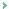Science is applied perception. Just as our everyday perception of reality leads us to form theories, for example, day follows night leads to a theory that day follows night, so science is really a theorising of what is already evident to observation - even by default, as in the effects observed. There can never be an original theory that is not based on the observation that preceded it. The effect of science calling its theories 'discoveries' or its appliances 'inventions' implies the coming to light of what was hidden. Moreover, just why it appears original is because at base scientific theory is for all its workability sceptical of its absolute objectivity in terms of 'what is'. To clinch this charater of 'what is' the discovery was attributed by the old guard of Galileo, Newton et al to being the work of God, since there is the absolute status of 'what is'. Nevertheless, without mention of God, one can say with certainty that whatever is the reality is what it is or 'what is', no matter the prevailing scientific theory. Given that everything is there in terms of 'what is', the role of man in discovery is superficial. The excitement of science is based on an illusion that 'what is' is its domain: on the contrary 'what is' is already fully present. Science should not conceive of itself as pioneering 'what is'; maybe then it will be cured of a dimension of futility in the state of the unknown, as is fully present in 'what is'. The old question as to whether one believes in God or not, actually implies that dependent on one's belief God does or does not exist. But 'what is' is unaffected by one's beliefs, and since all knowledge is ultimately in the form of 'what is', one cannot deny 'what is' as the only absolute knowledge there is. The absolute articulation of 'what is', on which all reality is ultimately based, must have a context in which it is knowledge: the absolute nature of 'what is' indicates an absolute context of knowledge, or God.

rdashby
09/08/2013 21:40 PM

Name *
Email ID
(will not be published)
Comment *
Characters
Verification Code*
Please fill the above code for verification.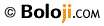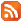RSS Feed Home | Privacy Policy | Disclaimer | Site Map